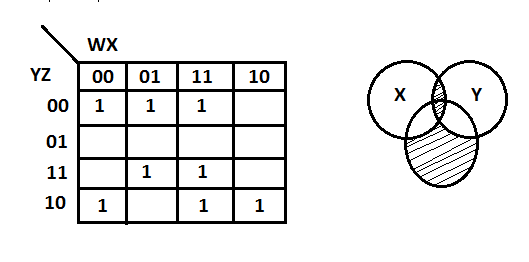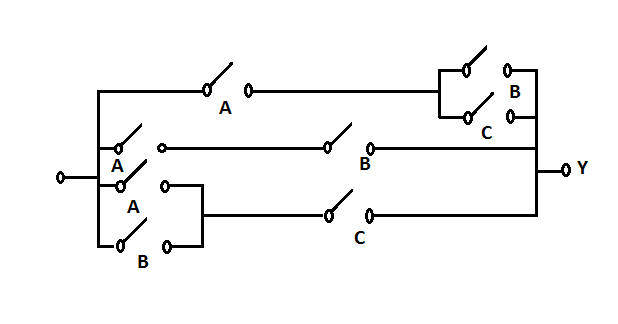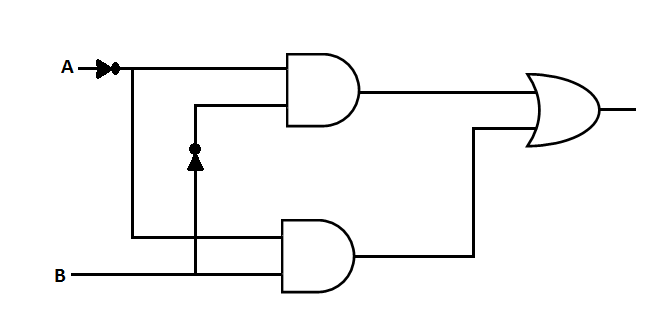# Digital Logic - Logic functions & Minimization

16:

Which of the following logic expression is incorrect?

 A. 1 ⊕ 0 = 1 B. 1 ⊕ 1 ⊕ 0 =1 C. 1 ⊕ 1 ⊕ 1 = 1 D. 1 ⊕ 1 = 0 Answer Report Discuss Option: B Explanation : Click on Discuss to view users comments. Write your comments here:
17:

In the following Karnaugh map, corresponding switching function in its minimal form isA. F( w , x , y , z ) = x' z' B. F( w , x , y , z ) = ( x' + z' ) ( w + z' )( x + y+ z ) C. F( w , x , y , z ) = x z  + w' z + x' y' z' D. F( w, x , y , z ) = x' z' + w z' w z' + xyz Answer Report Discuss Option: D Explanation : Click on Discuss to view users comments. Write your comments here:
18:

The minimum Boolean expression for the circuit is19:

Consider a function that is defined by the following truth table:

 A B C F(A, B, C ) 0 0 0 1 1 0 0 1 0 1 0 1 1 1 0 1 0 0 1 1 1 0 1 0 0 1 1 X 1 1 1 X

Which of the following statements about the minimal sum of products and minimal product of sums implementations is incorrect?

note that x represents don't care term

 A. They are logically equivalent because the don't cares are used in the same way B. They are not logically equivalent because the don't cares are used in the different ways C. They are not logically equivalent by definition D. They are logically equivalent by definition Answer Report Discuss Option: A Explanation : Click on Discuss to view users comments. Write your comments here:
20:

What is the Boolean expression for the circuit given below ?A. F( A , B ) = A' B + A' B' = A' B. F( A , B ) = A ⊕ B C. F( A , B ) = A' + B' D. F( A , B ) = A' B' Answer Report Discuss Option: A Explanation : Click on Discuss to view users comments. Write your comments here: